Prism for IIT JEE

Working and Types of Prism

A transparent object that has reflecting surfaces and that separates white light that passes through it into different colours is called a prism. It is a wedge-shaped body made from a refracting medium which is bounded by two plane faces inclined to each other with some angle. The angle included between these two faces is known as the angle of a prism or refracting angle and the two plane faces are known as refracting faces. Prism has the traditional triangular shape with a triangular base and rectangular sides. One of the most recognizable uses of the prism consists of dispersing a beam of white light into its component colours. This application is used by spectrographic component and refractometer. Prism can be made from any material that is transparent to the wavelength typical materials like glass, plastic, and fluorite. A dispersive prism can be used to break light into spectral colours. It can be used for reflecting the light, or to split light into the component with different polarization. Prisms have been used in, "folding" the system into a smaller space, "bending" light within a system, changing the orientation of an image, as well as combining or splitting optical beams with partial reflecting surfaces. We need multiple mirrors to achieve results similar to a single prism. Replacing mirror assemblies is perhaps the most useful application of prisms since they both fold or bend light and change image parity. One prism in the place of several mirrors reduces potential alignment error, increase accuracy and minimizes the size and complexity of the system.

How Prism Work:

When light moves from one medium to another, the speed of light changes. The change in speed is because the light to be refracted and to enter the new medium has a different angle. The degree of bending of the light’s path depends on the ratio between the refractive indices of two media, and on the angle that the incident beam of light makes with the surface.
Dispersion is the phenomenon in which the refractive index of any material varies with the colour of light used or the wavelength. As a result, the different colours of light are refracted differently and they leave the prism at different angles which creates an effect similar to a rainbow. Iridescent materials also show a similar separation, such as a soap bubble. Sometime prisms are used for the internal reflection on the surface. If light hits one of the surfaces at a sufficiently steep angle inside the prism, total internal reflection occurs and the light is reflected. Sometime prism is a useful substitute for a mirror.

Types of Prism:

Dispersive Prisms:

Dispersive prism is used to break up light into its spectrum colours because the refractive index depends upon the frequencies. The white light that enters in the prism is a mixture of different frequencies, each of which gets bend slightly differently. Blue light is always bent more than red light because the blue light is slower than the red light. An Amici prism is a type compound dispersive prism which is used in the spectrometers. It consists of two triangular prisms in contact with one another. Light enters in the first prism and is refracted at the first air-glass interface, refract again at the interface between two glasses and then exits the second prism.

Reflective Prism:

Reflective prism is used to reflect the light, in order to flip, rotate, invert, deviate or displace the light beam. They typically erect the image in binoculars or single lens reflex cameras. Many reflective prisms are used for total internal reflection to achieve high reflectivity. Porro prism is a type of reflective prism used in optical instruments to alter the orientation of an image. If an image is passing through Porro prism, the image rotates by 180° and exits from the opposite direction offset from its entry point. Porro prism is used in pairs forming a double Porro prism.

Beam-splitting Prism:

The beam-splitting prism is used for splitting the beam into two or more beams. Dichroic prism is a type of beam-splitting prism. It splits the light beam into two beams with differing wavelength. When two dichroic prisms combine to split an image into three colours, typically as red, green, blue of the RGB colour model, it is known as a trichroic prism. Dichroic prisms is constructed of one or more glass prism with dichroic optical coatings that selectively disseminate or send backlight and is dependent on the light wavelength.

Polarizing Prism:

A beam of light can be split into components of varying polarization by the polarizing prism. Polarizing prism is made of a birefringent crystalline material. Nicol prism is a type of polarizing prism. It is made from calcite crystal which is used to produce and analyze plane polarized light. Nicol prism removes one of the rays by total internal reflection, such as the ordinary ray is removed and the extraordinary ray is transmitted through the prism.

Deflecting Prism:

Deflecting prism is used to deflect a beam of light by a fixed angle. A pair of deflecting prism can be used for beam steering. The beam can be deflected into any desired angle within a conical by rotating the prisms. Risley prism pair is the most commonly founded implementation. Two wedge prisms can also be used to change the shape of a beam as an anamorphic pair. This is used to make a round beam from the elliptical output of laser diode.

Refraction through a glass prism:

Glass prism:

Let us take a glass prism as shown in the figure given below. It consists of two triangular ends and three rectangular faces. We know that the opposite faces of the triangular prism are not parallel to each other. In the diagram, M1 and M2 are the two faces of the prism where the refraction of light takes place. M1 and M2 are inclined to each other at an angle known as the angle of the prism. ∠AOC is an angle of the prism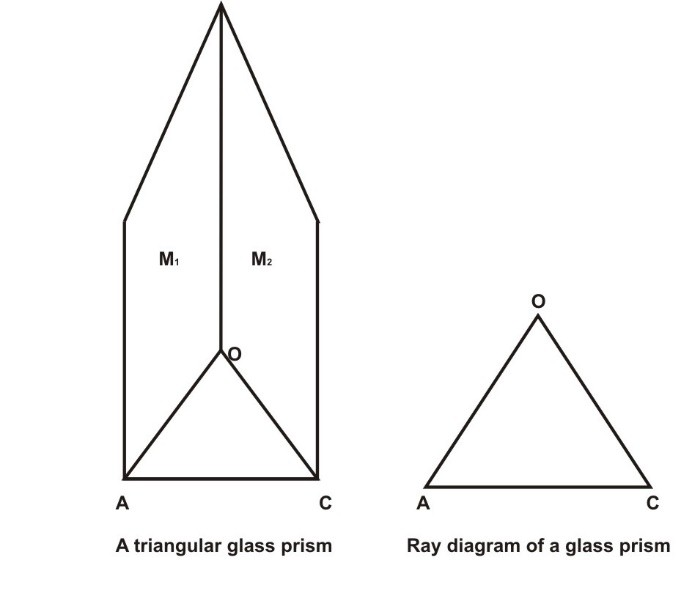The ray diagram of glass prism is like a triangle. Sometimes a glass prism is also called as a prism. The incident ray in a glass prism is not parallel to the emergent ray. It drifts from its original path by a certain angle. Now we study in detail how refraction takes place in a glass prism.

Reflection through glass prism:

When a light ray is passed through the prism it gets refracted twice. First when the light ray enters into the prism and second when the light ray exits from the prism. In the given figure there are two refracting surfaces i.e. OA and OB are not parallel to each other. So, the incident ray is also not parallel to the emergent ray. A glass prism OAB is made to stand on its base AB. Here the line JJ’ and KK’ are normal to the surfaces OA and OB respectively. LM is the incident light that falls on the surface of a prism. When the light ray is travelled from a rare medium to a denser medium, the light ray is bent toward normal QJ’ and follows the path QR inside the medium. QR is the emergent ray which bends towards the base of the prism. Now the refracted ray exits the prism at point R. At this point, the ray is again refracted because the ray QR is travelling from a denser medium to a rarer medium and the ray bends away from KR.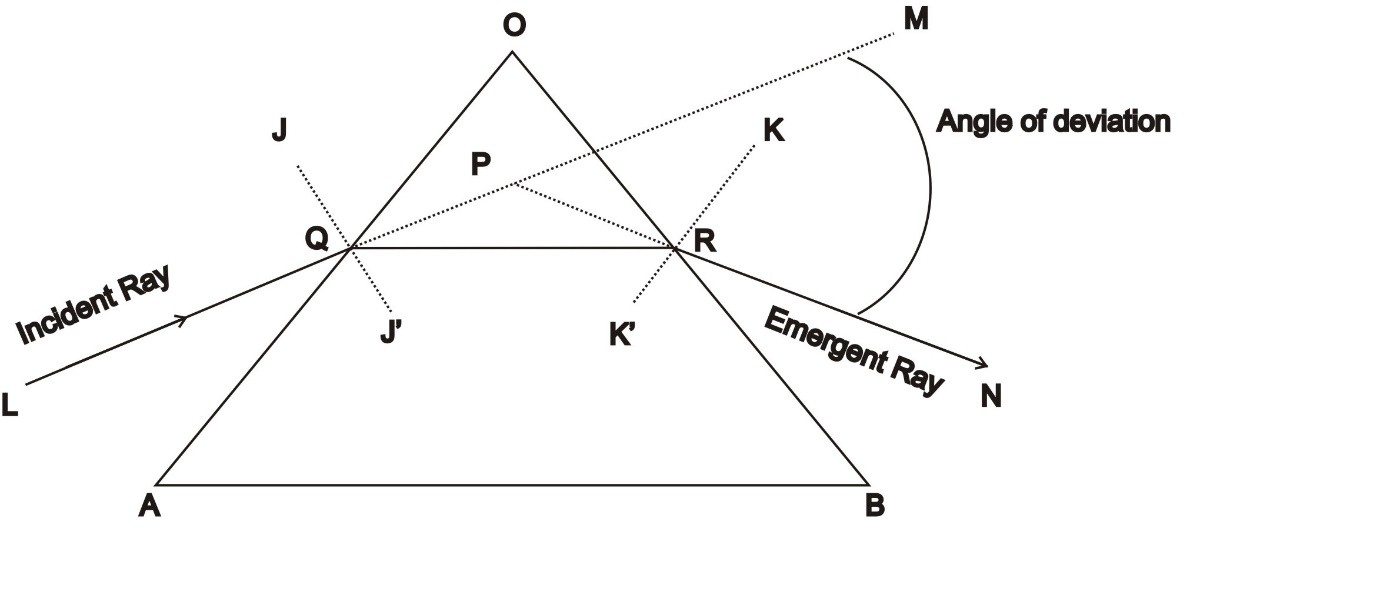So the conclusion is that when a light ray travels through a glass prism, it always bends towards the thicker part of the glass prism. We know that the surface of the prism is not parallel to each other so the emergent ray RN and incident ray LQ are also not parallel. Now, let us extend the incident ray LQ to point M. This extended line is the original path of the incident ray. So, the angle between the original path of the incident ray and the emergent ray is known as the angle of deviation.

Refractive Index of a prism: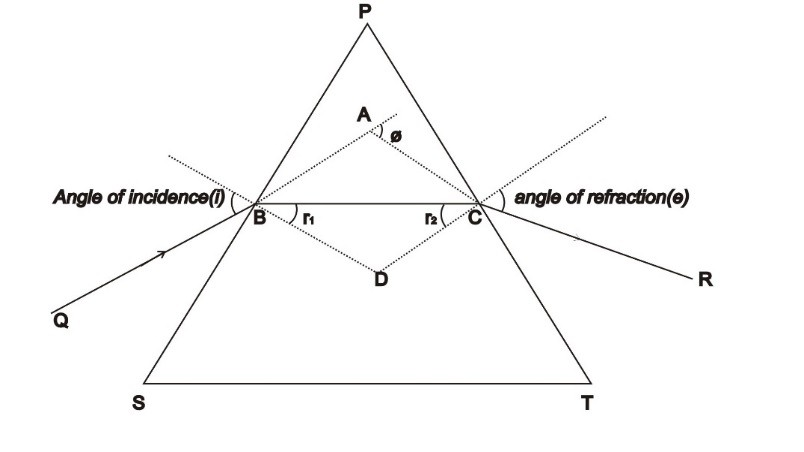Consider the prism given above. In the quadrilateral BDCP, at the vertices B and C both the angles are right angles. Therefore,
∠P + ∠BDC = 180° ………… (1)
In ∆ BDC,
r+ r2 + ∠BDC = 180°……….. (2)
r+ r2 = P …….. (3)

Now, as the sum of deviation at the two surfaces, we can define the total deviation ∅.
∅ = (i - r1) + (e - r2
∅ = i + e – A ………….. (4)
So, the conclusion is that the angle of deviation depends on the angle of incident.
If the deviation is negligible, the refracted ray becomes parallel to the base of the prism. A minimum deviation is denoted by Dmin which is equal to the angle of deviation, the angle of refraction (e) is equal to the angle of incident (i) i.e. i=e and r1=r2.
From equation (3),
2r = P
r = P/2
Similarly,
From equation (4),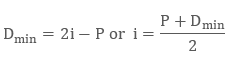The refractive index (µ) of the prism is,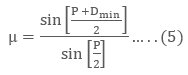Equation 5 determines the refractive index of the prism. For a prism, whose angle is negligible, Dmin is also very small.